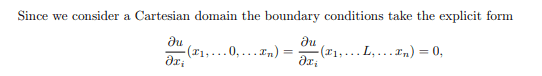# Mathematical Biology- Neumann BCs, Turing Analysis

• binbagsss

#### binbagsss

This is probably a stupid question but I have Neumann BC boundary : ## \nabla u . \vec{n} =0## (same for ##v##)conditions for the following reaction-diffusion system on a [0,L_1]x[0,L_2]x...x...[0,L_n] n times in n dimensional space so ##u=u(x_1,...,x_n,t)## is a scalar I believe?

so that ## \nabla u . \vec{n} ## is a vector times a vector is a scalar,

my notes then say:and so, I'm confused how to argue, from, a summation of ##u_i## derivatives we conclude that each ##u_i## derivative must individually be zero? unless we are specifying ##n## different normal vectors, one for each surface (divided by two for the what would be a negative of this normal vector) ? so like ##(1,0...0)## ,...,(0,0,...1) ##

ahh this must be the case actually and i have misinterpreted the boundary condition?? thanks

#### Attachments

Last edited by a moderator:
You only have one boundary condition per surface. The boundary conditions state that the derivative in the normal direction of the boundary is zero. This takes different forms on each boundary